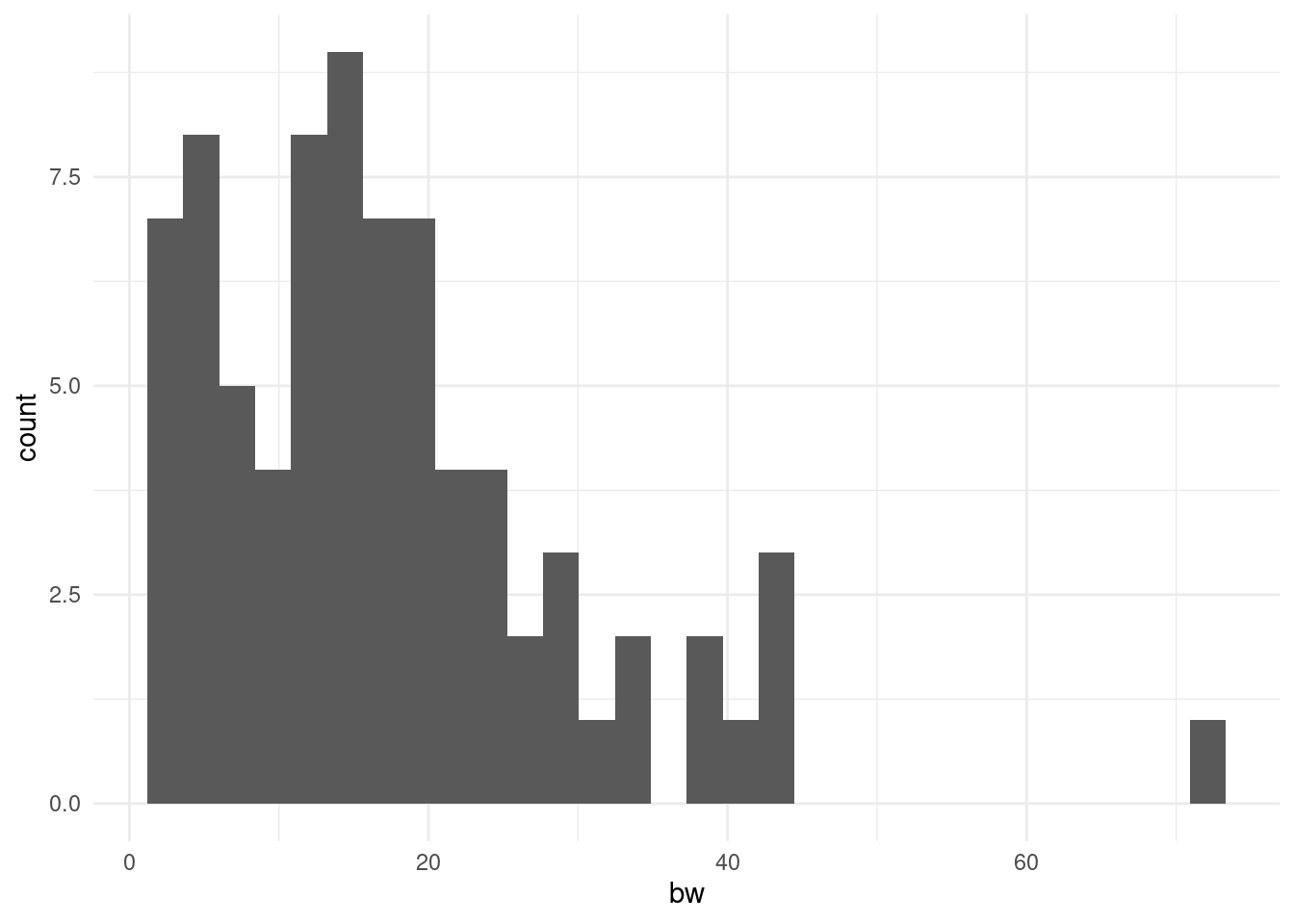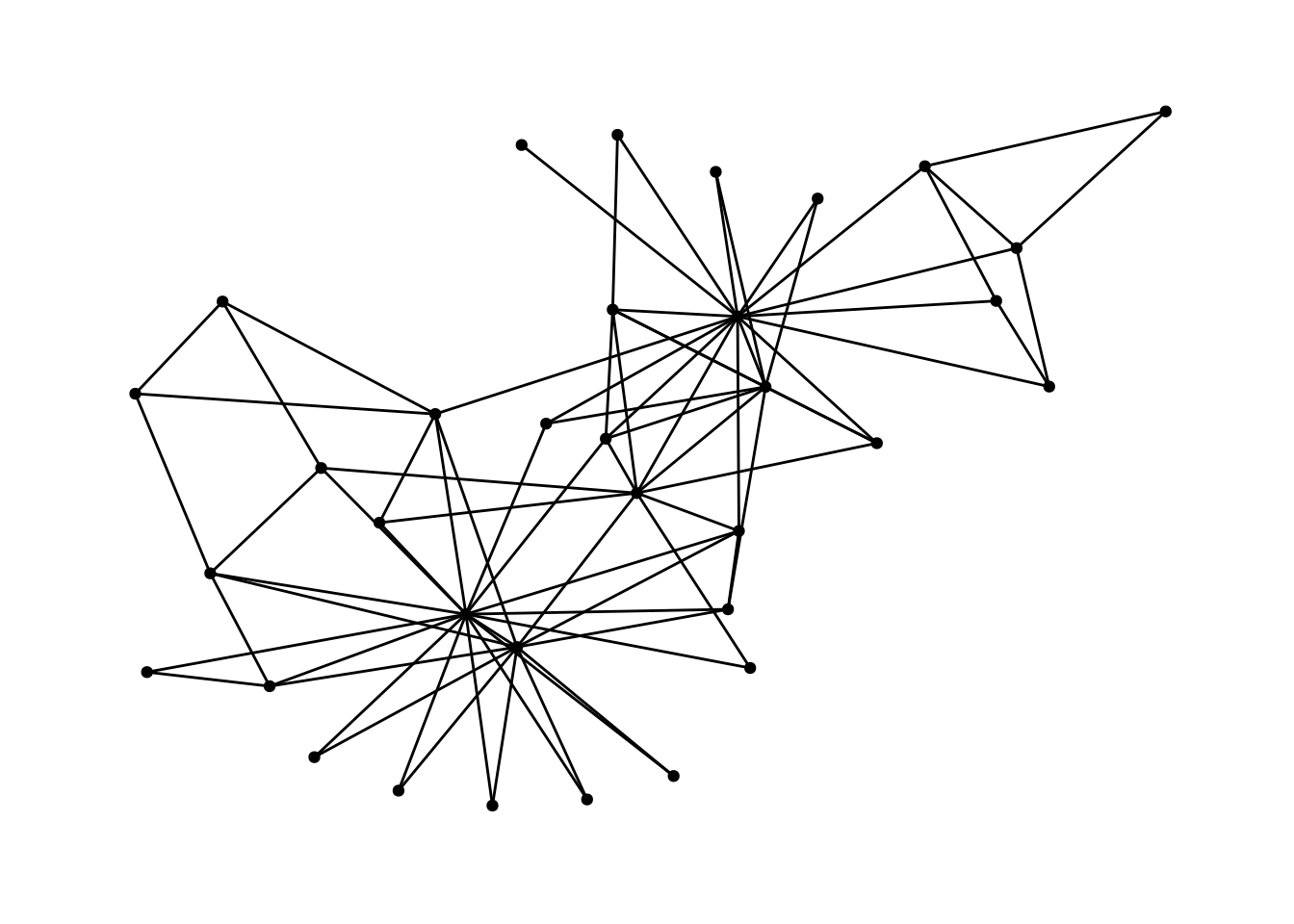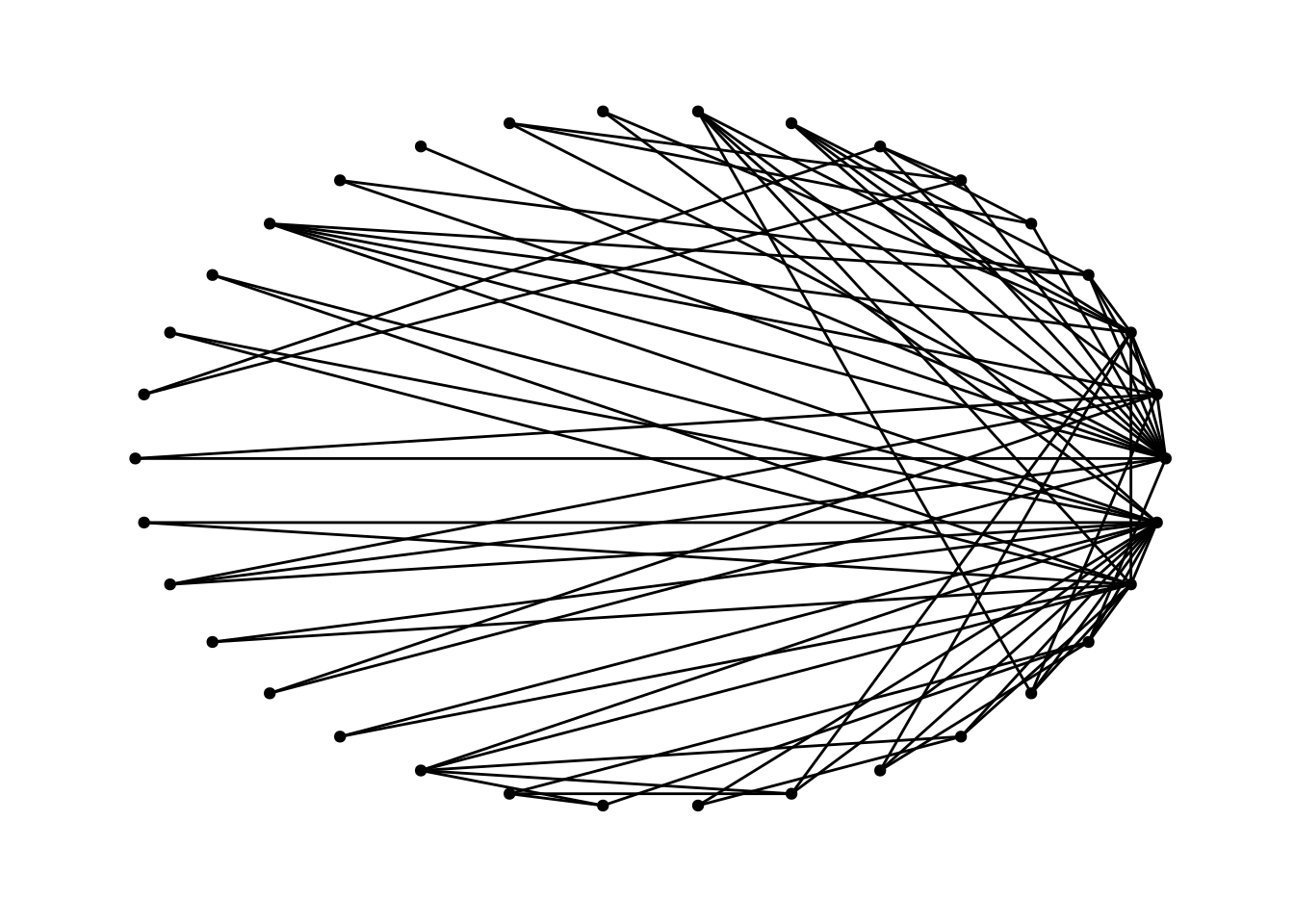R has a lot of great tools for working with tables (also called dataframes or tibbles). One really powerful and relatively intuitive set of tools is called the “tidyverse”. Tools in the tidyverse make assumptions about what data will look like – rows represent observations and columns represent variables about that observation.

The tidygraph package extends that paradigm to networks, by representing networks as two tables – a table of nodes and node attributes and a table of edges and edge attributes.

This lets you work with networks using many of the same tools that have been developed for working on other types of data.

# Getting to the data

## Activating a table

Because a network is really composed of two tables, we have to let R know which table we want to manipulate. This is done using `activate(nodes)` or `activate(edges)`.

For example, the code below activates the node table and then uses `mutate` (which we will talk more about further down) to create a variable called `degree` in the nodes table.

(Note that the code throughout this tutorial uses “pipes”. Pipes (`%>%`) let you express a sequence of operations, by taking the output of the previous operation and using it as the input of the next operation.)

``````create_notable('zachary') %>%
activate(nodes) %>%
mutate(degree = centrality_degree())``````
``````## # A tbl_graph: 34 nodes and 78 edges
## #
## # An undirected simple graph with 1 component
## #
## # Node Data: 34 x 1 (active)
##   degree
##    <dbl>
## 1     16
## 2      9
## 3     10
## 4      6
## 5      3
## 6      4
## # … with 28 more rows
## #
## # Edge Data: 78 x 2
##    from    to
##   <int> <int>
## 1     1     2
## 2     1     3
## 3     1     4
## # … with 75 more rows``````

Because the networks are just stored as data frames, that means that we can also do things like use `ggplot` to graph attributes of a network. This code below creates an edge attribute called `bw` which is a measure of edge betweenness, and then makes a histogram of the distribution of `bw`.

``````create_notable('zachary') %>%
activate(edges) %>%
mutate(bw = centrality_edge_betweenness()) %>%
ggplot() +
geom_histogram(aes(x=bw)) +
theme_minimal()``````
``## `stat_bin()` using `bins = 30`. Pick better value with `binwidth`.``# Plots

The companion library to `tidygraph` is ggraph. `ggraph` is a set of tools based on `ggplot2`. The key idea behind both `ggraph` and `ggplot2` is that you can build a plot by adding layers according to a “grammar of graphics” that let you add to and change things about the plot.

`ggraph` includes tons of really cool types of plots but for this tutorial I am going to focus on standard plots that show nodes as circles and edges as lines. There are three key components that should be part of any of these plots:

• layout
• nodes
• edges

## Layout

There are lots of layout options included with ggraph. You can let ggraph pick for you or you can look through some here and here.

Layouts are defined inside the `ggraph()` function, which has to be called before making any plot. The code below makes a tidy graph of the Zachary karate network using `create_notable('zachary')`. It then sets `'kk'` as the layout, and adds a layer for the nodes and a layer for the edges.

``````create_notable('zachary') %>%
ggraph(layout = 'kk') +
geom_node_point() +
geom_edge_fan()``````The following few plots show how changes the layout can really change the look and the interpretation of the plot. These all show the same data.

``````create_notable('zachary') %>%
ggraph(layout = 'circle') +
geom_node_point() +
geom_edge_fan()``````In this one, we get very fancy. This shows an ego network for node `3`. We will get to what some of the different commands in this code mean but like a good programmer what I really did was just find an example that was sort of like what I wanted to do, and played around with adding and changing things until I made it look like what I wanted.

``````create_notable('zachary') %>%
mutate(d = distances(.G(), to=3)) %>%
ggraph(layout = 'focus', focus = 3) +
geom_edge_fan() +
ggforce::geom_circle(aes(x0 = 0, y0 = 0, r = r), data.frame(r = 1:3), colour = 'grey') +
geom_node_point(aes(color = as.factor(d)), size = 3) +
coord_fixed() +
scale_color_viridis_d() +
labs(color='Distance from Node 3')``````